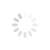## ABCD and THREE DRIVE pattern## One of the most basic harmonic patterns – ABCD pattern.

Here is how it works.You have AB and CD in the picture. These are called legs of the pattern. BC movement is a retraction. In this case:
• AB and CD are always going to be of equal length;
• Amount is time it takes to go from A to B is going to be the same as from C to D.

This pattern can be used to trace trend overturn using Fibonacci retracement. Here Fibonacci retracement on AB, the retracement BC should reach 0.618 level. Next, the line CD should be the 1.272 Fibonacci extension of BC. You can also use the percentage stated in the picture for calculations although it is better to simply use the rules.

## Moving on to the three drive pattern.

Three Drive pattern looks a lot like ABCD, but legs here are called drives and they usually have two corrective retracements. To spot them you are going to need all the same tools as with the previous entry. The main rules for three drive as follows:
• The time it would take the price to finish drive 2 should be equal to the time it takes to finish drive 3.
• The time to complete retracements A and B is equal.That is pretty much it. There is also a matter of Fibonacci retracement where point A is the 61.8% retracement of drive 1 and point B is the 0.618 retracement of drive 2. Then, drive 2 is the 1.272 extension of correction A and drive 3 is the 1.272 extension of correction B. Once that point is hit you need to get out!

### Related Items

Comments powered by CComment